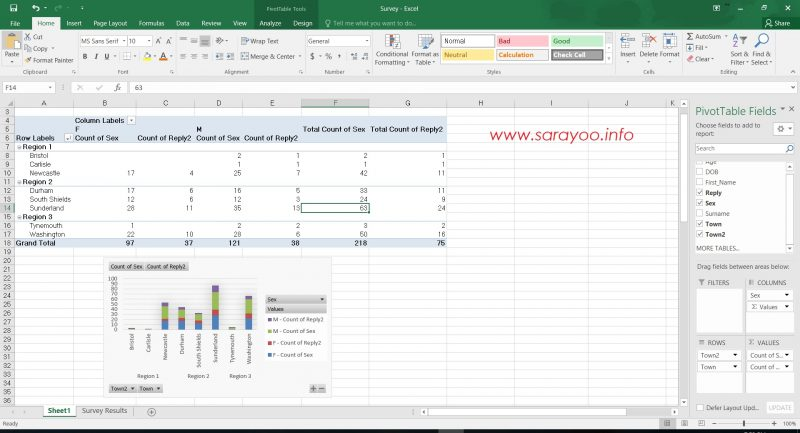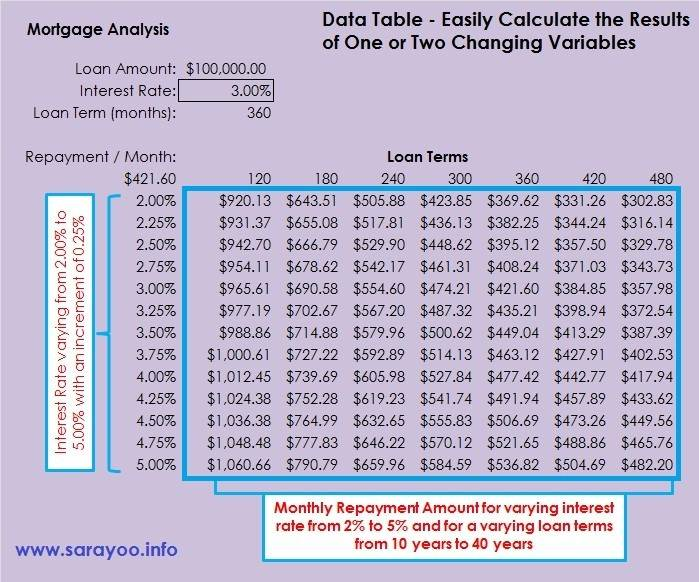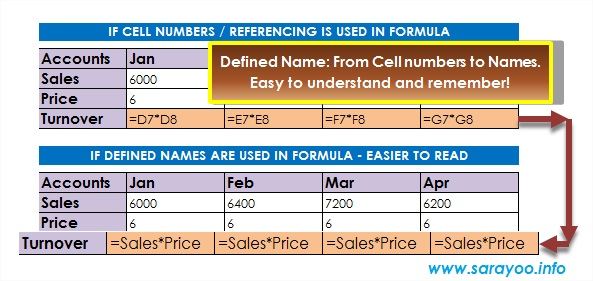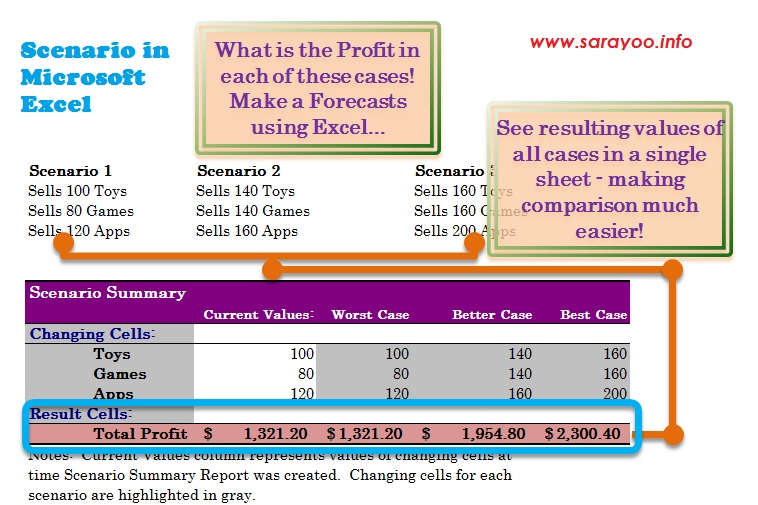# excel tips

## Create a PivotTable in Excel 2016 and Easily Analyze Large Data in your WorksheetRead: 19,791 One of the many great things about Microsoft Excel is that it allows you to manipulate large amount of data to make better, calculated business decisions. In the previous posts, we saw how we can create how we can use Data Tables, create Outlines, use Formulas, etc. in Excel. In this post, let us look at PivotTables. A… Read More »Create a PivotTable in Excel 2016 and Easily Analyze Large Data in your Worksheet

## Easily Calculate the Results of One or Two Changing Variables in Excel Using Data TablesRead: 27,772 Data tables are used to see how changing one or two variable data in a formula affects the result in a Microsoft Excel Worksheet. It creates a range of cells in a table form that shows the results when the variable data is applied in the calculation. Data table allows you to easily calculate multiple results in a… Read More »Easily Calculate the Results of One or Two Changing Variables in Excel Using Data Tables

## How to Use Names in Excel Formula and Make it Easier to UnderstandRead: 13,838 Usually we use cell numbers in formulas whenever we work on an Excel spreadsheet. Remembering each cell number and what it contains can be really hard. However, there is an easy way – you can use names in formulas instead of cell numbers. For example: imagine that cell C10 contains Price and cell D10 contains Quantity and you… Read More »How to Use Names in Excel Formula and Make it Easier to Understand

## How to Create Scenarios in MS Excel to Make Predictions and ForecastsRead: 11,208 There may be times when we need to experiment with several different values in an Excel Spreadsheet to make predictions and forecasts. For example, you are selling various products. Assume that you have a profit target to meet and you want to see how much profit you will make if you sell x number of each product. You… Read More »How to Create Scenarios in MS Excel to Make Predictions and Forecasts

## How to Switch Data From Columns to Rows or Rows to Column in Excel Quickly by Using Transpose DataRead: 7,666 In my previous post, we looked at how to create conditional formatting in order to highlight or make some cells standout from other cells based on it’s values. Today, I am going to show you how to re-arrange data in Excel from columns to rows and rows to columns easily by using transpose data feature. What Does Transposing… Read More »How to Switch Data From Columns to Rows or Rows to Column in Excel Quickly by Using Transpose Data

## Conditional Formatting in Excel 2010: Analyze and understand the Data at a GlanceRead: 7,890 Microsoft Excel is one of the very advanced spreadsheet applications that lets you easily create and manage complex spreadsheets. Imagine having a spreadsheet with large amount of data. Making some sense out of this data is a big task by itself. Scanning through rows and columns to read and interpret the data can be really difficult. For example,… Read More »Conditional Formatting in Excel 2010: Analyze and understand the Data at a Glance

## Solve Excel Error: There was a problem sending the command to the programRead: 6,976 You may have encountered the following error while trying to open a Excel document in Microsoft Excel 2010 or 2007, that says, “There was a problem sending the command to the program” which will prevent you from opening any excel file. You can resolve this error by following the steps below;   In Excel 2010 Step 1: Open… Read More »Solve Excel Error: There was a problem sending the command to the program